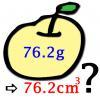#### You may also like### Far Horizon

An observer is on top of a lighthouse. How far from the foot of the lighthouse is the horizon that the observer can see?### Data Matching

Use your skill and judgement to match the sets of random data.### More or Less?

Are these estimates of physical quantities accurate?

# Genetics

##### Age 14 to 16Challenge Level

The boy who suffers from the disease must have inherited the alleles causing that disease from his parents. Since, however, both parents were healthy, we conclude that the disease-causing allele is recessive and that both parents are carriers.

In particular, this means that both parents have a copy of the "bad" gene and a copy of the "good" gene but since the "good" gene is dominant, both parents are healthy.

If we suppose that the disorder X is X - linked, this would mean that Allan, the father, would need to have a copy of the "good" gene, since he is healthy. Therefore, the boy who has the disease, must have inherited an X chromosome with the "bad" gene from Beth, his mother. As Beth is healthy, she must have a dominating "good" gene as well as the recessive "bad" gene. This is consistent with the data we are given, so the disorder X can be X - linked.

So, the gene determining whether a person has the disease X or not lies on one of the 22 other chromosomes. We also know that both parents have a copy of the dominant "good" gene and a copy of the recessive "bad" gene. In order for an offspring to suffer from the disease, they need to inherit the "bad" gene from both parents.

If we denote by G the "good" gene and by g the "bad" gene, we see that the possible combinations of genes in the offspring are: GG, Gg, gG and gg. Hence, the only case in which the "bad" gene is inherited from both parents (gg) has a probability of $\frac{1}{4}$ of occurring.

Therefore, each child has a chance of $\frac{1}{4}$ of having the disease.

Moreover, each child has a chance of $\frac{1}{2}$ of being a boy or a girl. So, in order to have a family of 5 children, with 3 healthy girls, 1 healthy boy and 1 unhealthy boy, we have a probability of:

$$P = P(\textrm{3 girls and 2 boys})\cdot P(\textrm{3 healthy girls})\cdot P(\textrm{1 healthy and 1 unhealthy boy})$$

We will calculate all these probabilities separately.

The probability of having 3 girls and 2 boys out of 5 children is (using the binomial distribution)

$$P(\textrm{3 girls and 2 boys})=\binom{5}{3}\cdot \left(\frac{1}{2}\right)^5 = \frac{5}{16}$$

Moreover, the probability of each girl being healty is $\frac{3}{4}$, hence the probability of all three girls being healthy (since each birth is independent) is

$$P(\textrm{3 healthy girls}) = \left(\frac{3}{4}\right)^3 = \frac {27}{64}$$

We also want that from the two boys, one is healthy and one is not. The probability of this happening is (using the binomial distribution again)

$$P(\textrm{1 healthy and 1 unhealthy boy}) = \binom{2}{1} \cdot \frac{1}{4} \cdot \frac {3}{4} = \frac {3}{8}$$

So, multiplying all of the above, we get our desired expression for the total probability of having 3 healthy girls, 1 healthy boy and 1 unhealthy boy:

$$P = \frac{5}{16} \cdot \frac{27}{64} \cdot \frac {3}{8} = \frac{405}{2048}$$

Now, suppose that the disease is in fact X - linked. Then, we denote by X the X-chromosome carrying the "good" gene, by x the X-chromosome carrying the "bad" gene, and by Y the Y-chromosome. As we have explained before, the genotype of Allan is XY and the genotype of Beth is Xx.

Therefore, each of the daughters Allan and Beth might have will be certainly healthy, since she will inherit the "good" X chromosome from Allan, which is dominant (we recall that every girl inherits her father's only X chromosome).

Thus, $$P (\textrm{3 healthy girls}) = 1$$

Now, for the two boys, each of them will inherit their father's Y chromosome, and either X or x from their mother, with equal likelihood. Hence, the probability of a boy being unhealthy is $\frac{1}{2}$ and hence

$$P (\textrm{1 healthy and 1 unhealthy boy}) = \binom{2}{1} \cdot \left(\frac{1}{2}\right)^2 = \frac{1}{2}$$

Again, we need to multiply this with the probability of the family actually having 3 girls and 2 boys, and so we obtain

$$P = \frac{5}{16} \cdot 1 \cdot \frac{1}{2} = \frac{5}{32}$$

We now compare the probabilities associated with the two different cases, and we note that

$$\frac{405}{2048} > \frac{5}{32}$$

This, however, does not mean that the disease is more likely not to be X - linked. It simply means, that if the disease is not X - linked, then it is more likely that a family with 3 girls and 2 boys will have 3 healthy girls, 1 healthy boy and 1 unhealthy boy.